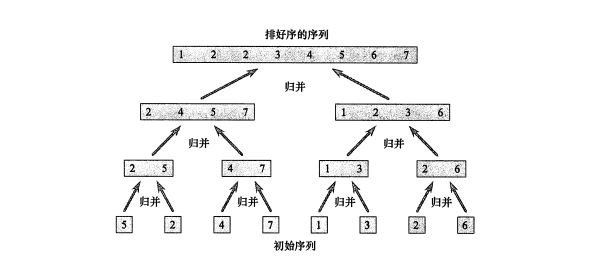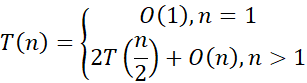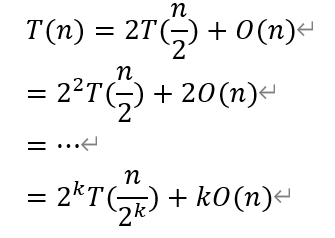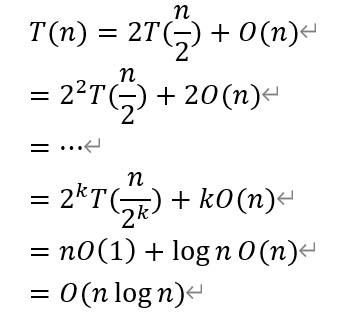# 算法

## 为什么要用算法

• 为了走出迷宫，你可能需要DFS，即深度优先搜索算法来寻找出路。
• 为了找到最短路径，你可能要用到A*算法来高效查找。
• 为了将一串数字排序，你需要用更快的快速排序算法，而不是一个一个比较。

# 算法效率

## 渐进时间复杂度

``````for(int i=0;i<n;i++){
for(int j=0;j<n;j++){
swap(a[i],a[j]);
}
}``````

# 分治法

## 归并排序``````void sort(int *list, int *result, int left, int right) {
//如果left不小于right，则直接退出
if (left >= right) return;
//将数组从中间切割,mid为切割位置
int mid = ((right - left) >> 1) + left;
//切割后的两个数组的起始和终止位置
int left_1 = left, right_1 = mid;
int left_2 = mid + 1, right_2 = right;
//将切割后的数组排序
sort(list, result, left_1, right_1);
sort(list, result, left_2, right_2);
int i = left;
//合并排序后的数组
while (left_1 <= right_1 && left_2 <= right_2){
result[i++] = list[left_1] < list[left_2]
? list[left_1++]
: list[left_2++];
}
while (left_1 <= right_1){
result[i++] = list[left_1++];
}
while (left_2 <= right_2){
result[i++] = list[left_2++];
}
//赋值
for (i = left; i <= right; i++){
list[i] = result[i];
}
}``````

## 时间复杂度由于迭代到最后,2的k次方应该等于n，所以可以直接使用logn来替换k，因此完整推导过程如下# 评论

### 评论列表

##### 微信小程序##### QQ小程序